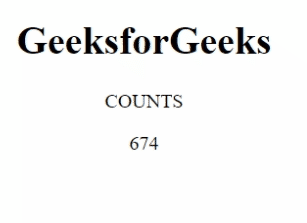GeeksforGeeks App
Open AppBrowser
Continue

# How to make animated counter using JavaScript ?

In this article, we will create an animated counter using JavaScript and basic HTML for designing.

An animated counter is used to display the counts in an animation starting from 0 and ending at a specific number. This is generally used by many websites to make a web page more attractive.

Approach:

• In the HTML body tag, specify the div tag with an id.
• Add JavaScript code to the script tag.
• Use the setInterval() method to execute the update function which will increment the count value.
• Get the id using the getElementById() method and use the innerHTML property to display the count.
• If the count value reached the required count then stop executing the code using the clearInterval() method.

The setInterval() method repeats a given function at every given time interval and this method executes until clearInterval() is called.

Note: Please refer to the above-mentioned article links for a better understanding.

Example 1: In the following code, we have taken variable “counts” which executes the setInterval() method which calls the updated() function. Once the “upto” variable value reaches the value of 1000, the clearInterval() method is executed. We have incremented the value of the counter value from 0 to 1000.

## HTML

 ```<``html` `lang``=``"en"``>` `<``body` `style``=``"text-align:center"``>``    ``<``h1``>GeeksforGeeks``    ``<``p``>COUNTS``    ``<``div` `id``=``"counter"``>``        ````    ``` `    ``<``script``>``        ``let counts = setInterval(updated);``        ``let upto = 0;``        ``function updated() {``            ``let count = document.getElementById("counter");``            ``count.innerHTML = ++upto;``            ``if (upto === 1000) {``                ``clearInterval(counts);``            ``}``        ``}``    ```````

Output:Example 2: Decrement the counter value from 1000 to 0 using JavaScript. We have added some CSS styles under the style tag in the body tag to make the webpage more attractive.

## HTML

 ```<``html` `lang``=``"en"``>``<``head``>``    ``<``style``>``        ``body {``            ``background-color: green;``        ``}` `        ``#counter {``            ``color: white;``        ``}``    ````` `<``body` `style``=``"text-align:center"``>``    ``<``h1``>GeeksforGeeks``    ``<``p``>COUNTS DECREMENT``    ``<``div` `id``=``"counter"``>``        ````    ``` `    ``<``script``>``        ``let counts = setInterval(updated);``        ``let upto = 1000;``        ``function updated() {``            ``let count = document.getElementById("counter");``            ``count.innerHTML = --upto;``            ``if (upto === 0) {``                ``clearInterval(counts);``            ``}``        ``}``    ```````

Output:My Personal Notes arrow_drop_up
Related Tutorials